# Feature Selection using Scikit-Learn in Python

Learn how to use Scikit-Learn library in Python to perform feature selection with SelectKBest, random forest algorithm and recursive feature elimination (RFE).
7 min read · Updated jul 2022 · Machine Learning

Disclosure: This post may contain affiliate links, meaning when you click the links and make a purchase, we receive a commission.

Many steps are involved in the data science pipeline, going from raw data to building an optimized machine learning model for the given task. However, data processing is the step that requires the most effort and time, and which has a direct influence and impact on the performance of the models later on.

In this article, we will focus on how to apply some feature selection on our dataset which represents a core aspect of the data preprocessing phase. But before diving into coding and implementing the different techniques used for these tasks, let us first define what we mean by feature selection.

Feature selection is the process of choosing a subset of features from the dataset that contributes the most to the performance of the model, and this without applying any type of transformation on it.

The dataset we will use is the Heart Disease Prediction dataset from Kaggle and you can directly work on that using the Kaggle Kernel VM, or you can download it to your local machine.

The following is the command for installing the required libraries for this tutorial:

``\$ pip3 install numpy pandas matplotlib sklearn``

Preprocessing

We will first load our dataset into a dataframe format using pandas. It is composed of 13 features plus the label and there are 270 rows.

``````import numpy as np
import pandas as pd
import matplotlib.pyplot as plt
from sklearn.model_selection import train_test_split
from sklearn.preprocessing import MinMaxScaler
from sklearn.feature_selection import SelectKBest,chi2,RFE
from sklearn.ensemble import RandomForestClassifier

print(df.shape)

The above code imports the necessary libraries and reads the dataset CSV file from `data` folder, to follow along, you need to create `data` folder as well, you can change the path to the location of dataset file in your machine.

Below is the output:

``(270, 14)``From here we can clearly observe that there are no null values, so we can directly start working on our dataframe without performing some null values cleaning.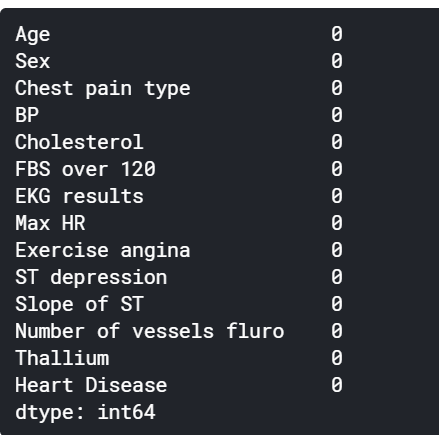We will store the label column into a separate variable and drop it entirely (hence, the use of `inplace=True`) from the dataframe. This step is important when we will be dividing our dataset into training and testing in addition to when we will be fitting them to our model.

``````label = df["Heart Disease"]
df.drop("Heart Disease", axis=1, inplace=True)``````

It is important to always check how imbalanced our dataset might be, since a big imbalance ratio between the minority and majority class will negatively affect the model in a sense where it will predict naively only the majority class. For our case however the imbalance ratio is only `1.25` which it is not big.

``````print(label.value_counts())
label.value_counts().plot(kind="bar")``````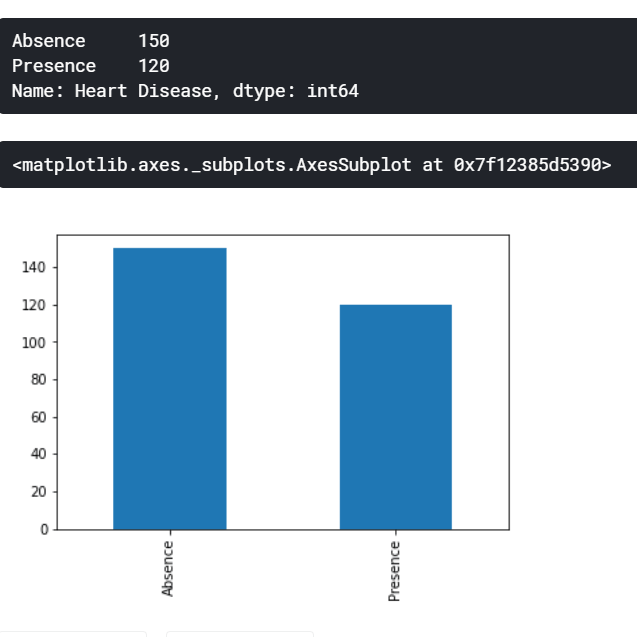Our dataset has features that follow a categorical nature. However, while printing the data type of those columns, we observe that they are considered as integers and this might make our model to treat them as continuous values despite being discrete by nature.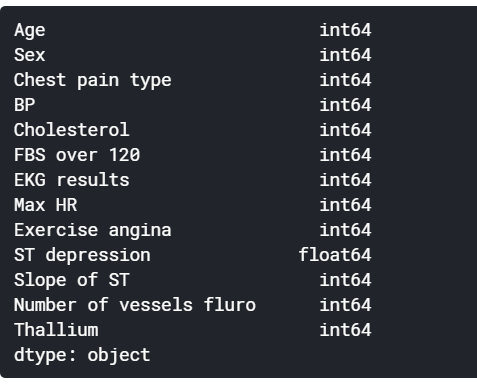For this reason we will change explicitly their data type to categorical using `astype()` pandas method.

``````categorical_features = ["Sex", "Chest pain type", "FBS over 120", "EKG results", "Exercise angina", "Slope of ST", "Number of vessels fluro", "Thallium"]
df[categorical_features] = df[categorical_features].astype("category")``````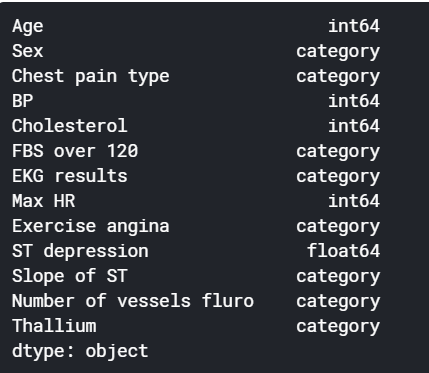We will now scale our continuous features using `MinMaxScaler`, it is a type of normalization where the values will range between 0 and 1 and the equation is defined by `X_Norm = (X - X_Min) / (X_Max - X_Min).`

``````continuous_features = set(df.columns) - set(categorical_features)
scaler = MinMaxScaler()
df_norm = df.copy()
df_norm[list(continuous_features)] = scaler.fit_transform(df[list(continuous_features)])``````

Feature Selection Using Chi-Square

A chi-square test is used in statistics to test the independence of two events. Given the data of two variables, we can get observed count O and expected count E. Chi-Square measures how expected count E and observed count O deviates each other.

In feature selection, and since chi2 tests the degree of independence between two variables, we will use it between every feature and the label and we will keep only the `k` number of features with the highest chi2 value, because we want to keep only the features that are the most dependent of our label. We will important both `SelectKBes`t and `chi2` from `sklearn.feature_selection` module. `SelectKBest` requires two hyperparameter which are:

• `k`: the number of features we want to select.
• `score_func`: the function on which the selection process is based upon.
``X_new = SelectKBest(k=5, score_func=chi2).fit_transform(df_norm, label)``

Feature Selection Using Recursive Feature Elimination (RFE)

From sklearn Documentation: The goal of recursive feature elimination (RFE) is to select features by recursively considering smaller and smaller sets of features. We will be using `sklearn.feature_selection` module to import `RFE` class as well. `RFE` requires two hyperparameters:

• `n_features_to_select`: the number of features we want to select.
• `estimator`: Which type of machine learning model will be used for the prediction in every iteration while recursively searching for the appropriate set of features.
``````rfe = RFE(estimator=RandomForestClassifier(), n_features_to_select=5)
X_new = rfe.fit_transform(df_norm, label)
X_new``````

Feature Selection Using Random Forest

Tree-based machine learning algorithms like `DecisionTreeClassifier` or their ensemble learning equivalent `RandomForestClassifier` uses a set of trees which contains nodes resulting from splitting. The main aim of those splits is to decrease impurity as much as possible by using impurity measures like entropy and gini index. Those tree-based models can calculate how much important a feature is by calculating the amount of impurity decrease this feature will lead to.

``````clf = RandomForestClassifier()
clf.fit(df_norm, label)
# create a figure to plot a bar, where x axis is features, and Y indicating the importance of each feature
plt.figure(figsize=(12,12))
plt.bar(df_norm.columns, clf.feature_importances_)
plt.xticks(rotation=45)``````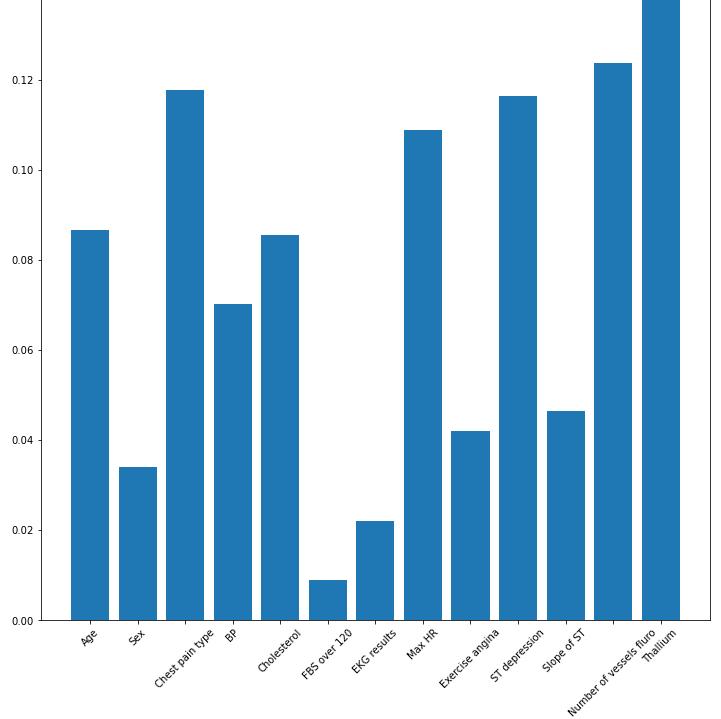The above histogram shows the importance of each feature. In our case, Thallium and number of vessels fluro are the most important features, but most of them have importance, and since that's the case, it's pretty much worth feeding these features to our machine learning model.

Now that you have selected the best features, you can easily use any sklearn classifier model and feed `X_new` array and see if it impacts accuracy of the full features model.

Conclusion

Using such techniques for feature selection varies from one problem to another and also from a feature to another depending on their type being categorical or continuous.

In addition, the number of features to select, can be answered by following an iterative approach until the `k` (in `SelectKBest`) converges and the machine learning performance is not increasing too much.

In this article, we have learned how to:

• Use chi2 to select the features with a high dependence on the label.
• Use RFE to recursively find the optimal set of features given an estimator.
• Use tree-based machine learning methods like Random Forest to display the features that help to reduce as much as possible the impurity while splitting the nodes.

Finally, I've collected some useful resources and courses for you for further learning, I highly recommend the following courses:

Happy Learning ♥

Sharing is caring!

### Comment panel